# Chapter 8 Arrays and Strings Objectives In this

• Slides: 57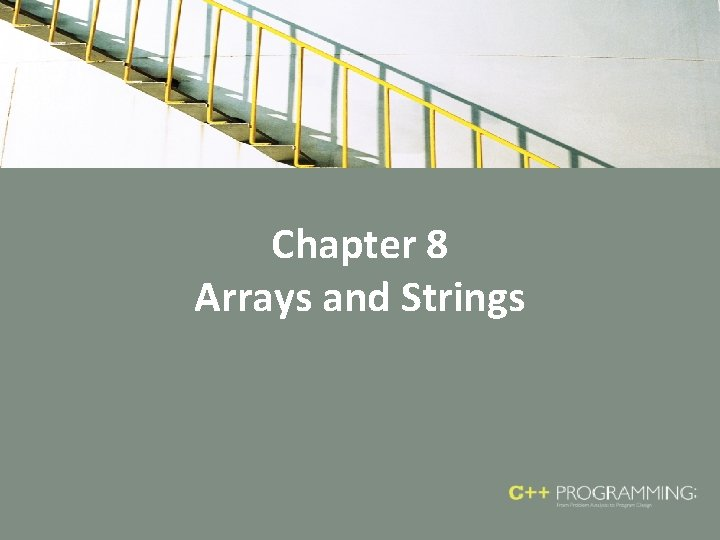Chapter 8 Arrays and StringsObjectives • In this chapter, you will: – Learn the reasons for arrays – Explore how to declare and manipulate data into arrays – Understand the meaning of ‘‘array index out of bounds’’ – Learn how to declare and initialize arrays – Become familiar with the restrictions on array processing C++ Programming: From Problem Analysis to Program Design, Seventh Edition 2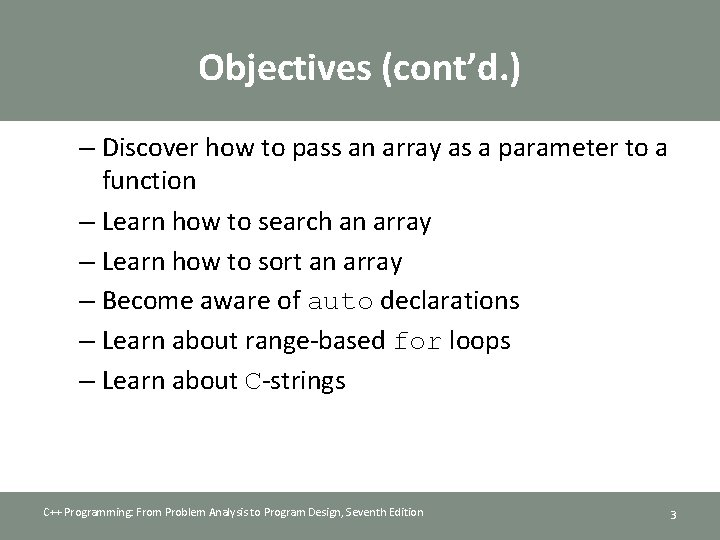Objectives (cont’d. ) – Discover how to pass an array as a parameter to a function – Learn how to search an array – Learn how to sort an array – Become aware of auto declarations – Learn about range-based for loops – Learn about C-strings C++ Programming: From Problem Analysis to Program Design, Seventh Edition 3Objectives (cont’d. ) – Examine the use of string functions to process Cstrings – Discover how to input data into—and output data from—a C-string – Learn about parallel arrays – Discover how to manipulate data in a twodimensional array – Learn about multidimensional arrays C++ Programming: From Problem Analysis to Program Design, Seventh Edition 4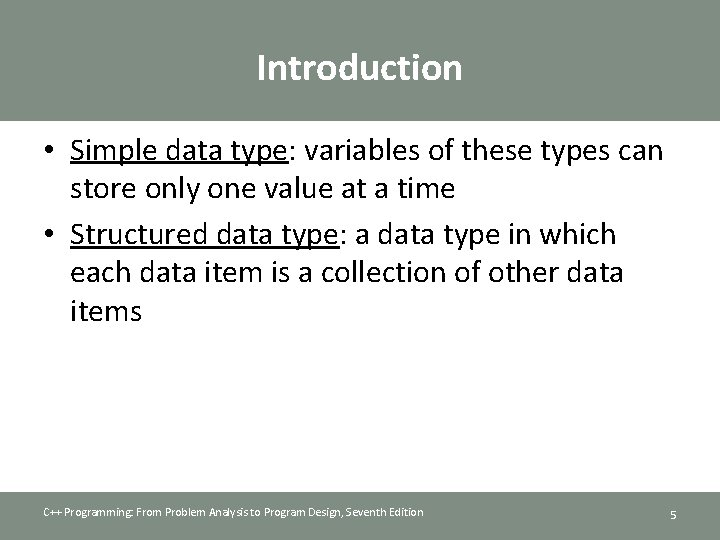Introduction • Simple data type: variables of these types can store only one value at a time • Structured data type: a data type in which each data item is a collection of other data items C++ Programming: From Problem Analysis to Program Design, Seventh Edition 5Arrays • Array: a collection of a fixed number of components, all of the same data type • One-dimensional array: components are arranged in a list form • Syntax for declaring a one-dimensional array: • int. Exp: any constant expression that evaluates to a positive integer C++ Programming: From Problem Analysis to Program Design, Seventh Edition 6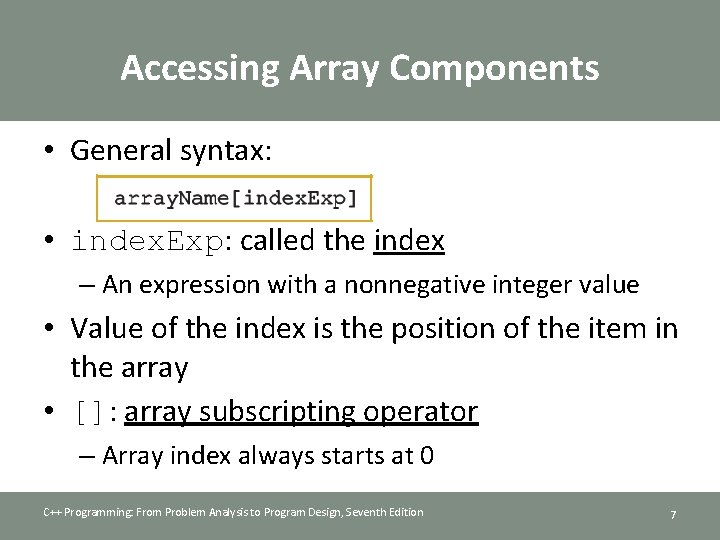Accessing Array Components • General syntax: • index. Exp: called the index – An expression with a nonnegative integer value • Value of the index is the position of the item in the array • []: array subscripting operator – Array index always starts at 0 C++ Programming: From Problem Analysis to Program Design, Seventh Edition 7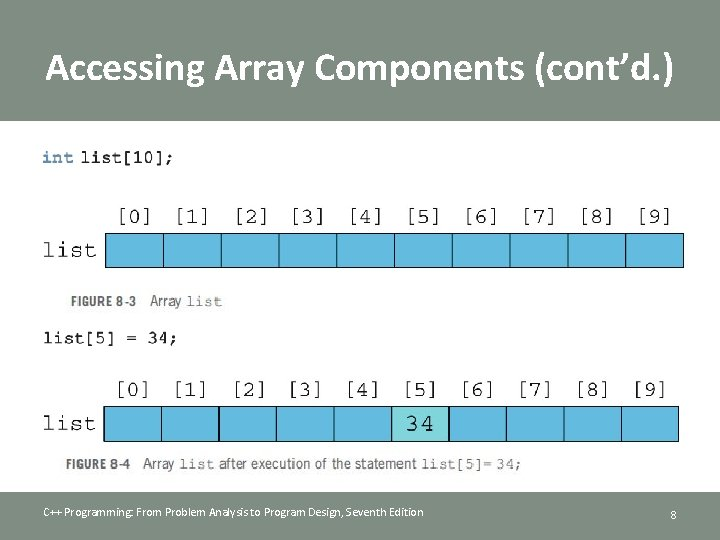Accessing Array Components (cont’d. ) C++ Programming: From Problem Analysis to Program Design, Seventh Edition 8Accessing Array Components (cont’d. ) C++ Programming: From Problem Analysis to Program Design, Seventh Edition 9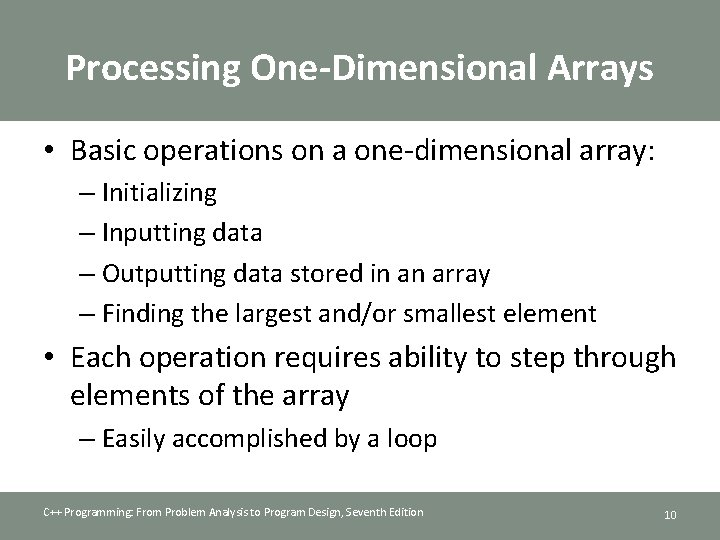Processing One-Dimensional Arrays • Basic operations on a one-dimensional array: – Initializing – Inputting data – Outputting data stored in an array – Finding the largest and/or smallest element • Each operation requires ability to step through elements of the array – Easily accomplished by a loop C++ Programming: From Problem Analysis to Program Design, Seventh Edition 10Processing One-Dimensional Arrays (cont’d. ) • Given the declaration: int list; int i; //array of size 100 • Use a for loop to access array elements: for (i = 0; i < 100; i++) cin >> list[i]; C++ Programming: From Problem Analysis to Program Design, Seventh Edition //Line 1 //Line 2 11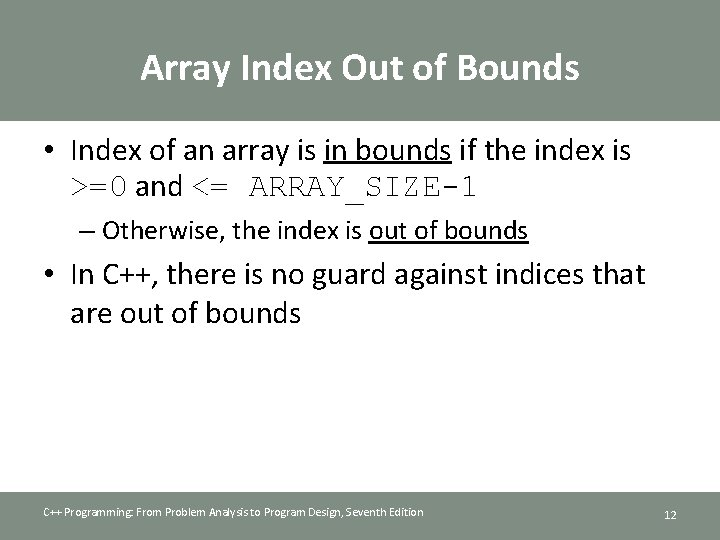Array Index Out of Bounds • Index of an array is in bounds if the index is >=0 and <= ARRAY_SIZE-1 – Otherwise, the index is out of bounds • In C++, there is no guard against indices that are out of bounds C++ Programming: From Problem Analysis to Program Design, Seventh Edition 12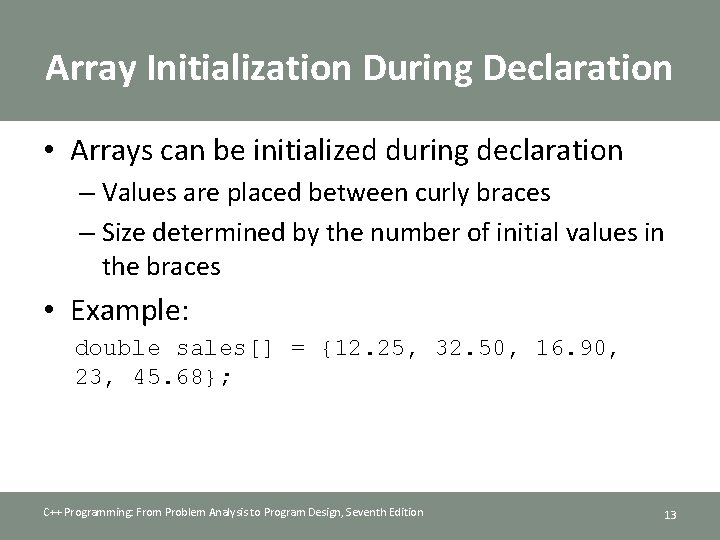Array Initialization During Declaration • Arrays can be initialized during declaration – Values are placed between curly braces – Size determined by the number of initial values in the braces • Example: double sales[] = {12. 25, 32. 50, 16. 90, 23, 45. 68}; C++ Programming: From Problem Analysis to Program Design, Seventh Edition 13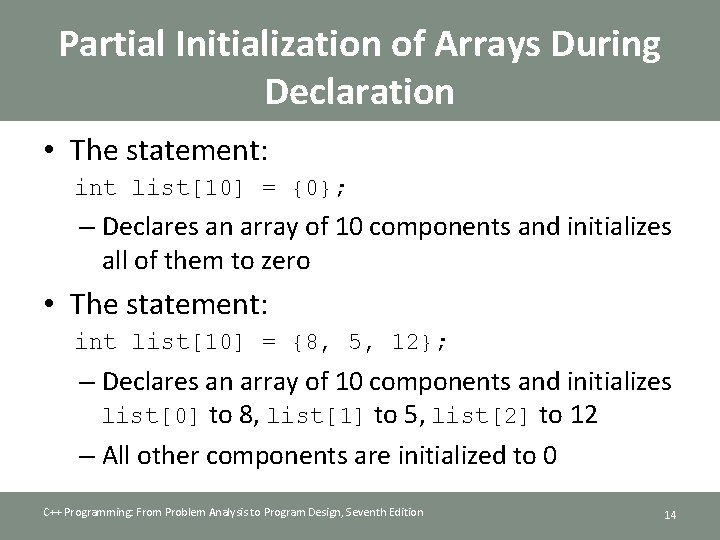Partial Initialization of Arrays During Declaration • The statement: int list = {0}; – Declares an array of 10 components and initializes all of them to zero • The statement: int list = {8, 5, 12}; – Declares an array of 10 components and initializes list to 8, list to 5, list to 12 – All other components are initialized to 0 C++ Programming: From Problem Analysis to Program Design, Seventh Edition 14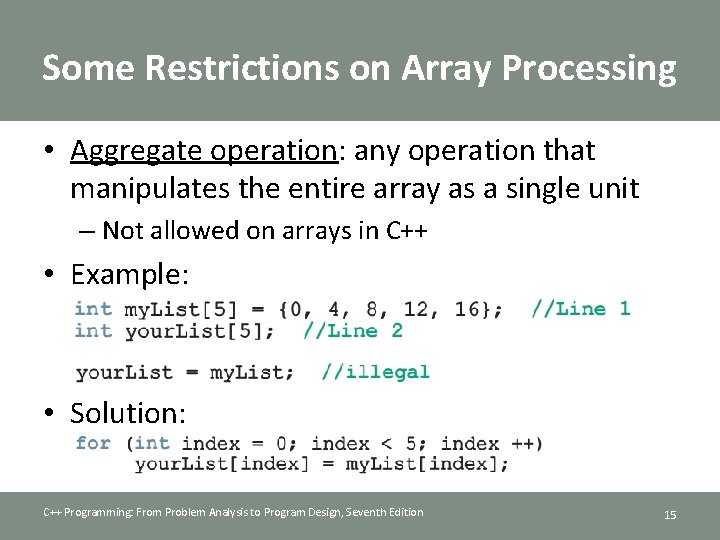Some Restrictions on Array Processing • Aggregate operation: any operation that manipulates the entire array as a single unit – Not allowed on arrays in C++ • Example: • Solution: C++ Programming: From Problem Analysis to Program Design, Seventh Edition 15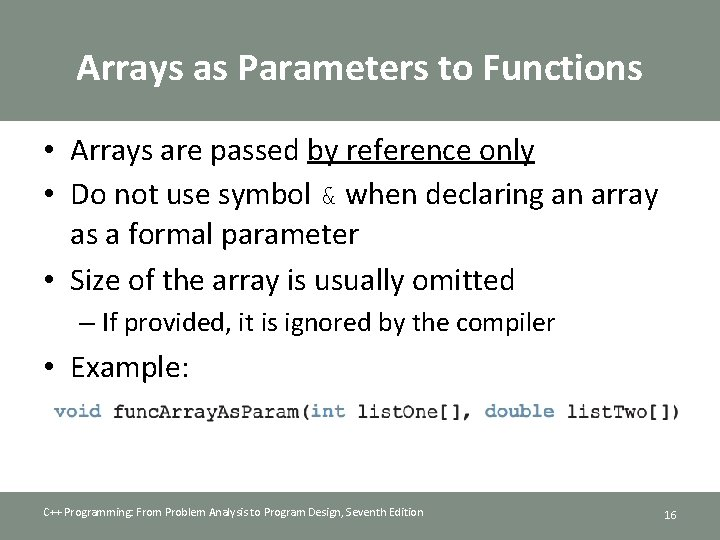Arrays as Parameters to Functions • Arrays are passed by reference only • Do not use symbol & when declaring an array as a formal parameter • Size of the array is usually omitted – If provided, it is ignored by the compiler • Example: C++ Programming: From Problem Analysis to Program Design, Seventh Edition 16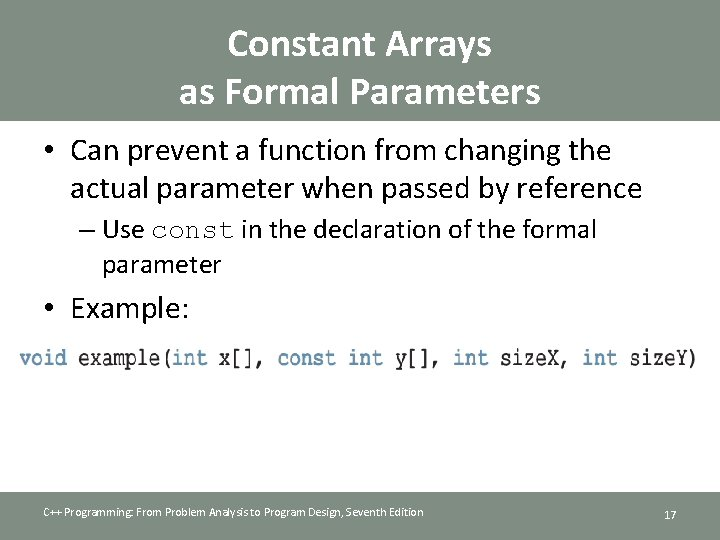Constant Arrays as Formal Parameters • Can prevent a function from changing the actual parameter when passed by reference – Use const in the declaration of the formal parameter • Example: C++ Programming: From Problem Analysis to Program Design, Seventh Edition 17Base Address of an Array and Array in Computer Memory • Base address of an array: address (memory location) of the first array component • Example: – If list is a one-dimensional array, its base address is the address of list • When an array is passed as a parameter, the base address of the actual array is passed to the formal parameter C++ Programming: From Problem Analysis to Program Design, Seventh Edition 18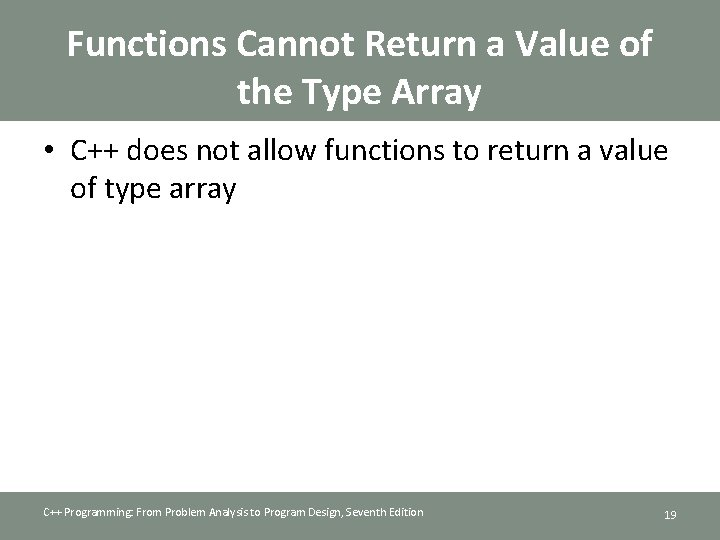Functions Cannot Return a Value of the Type Array • C++ does not allow functions to return a value of type array C++ Programming: From Problem Analysis to Program Design, Seventh Edition 19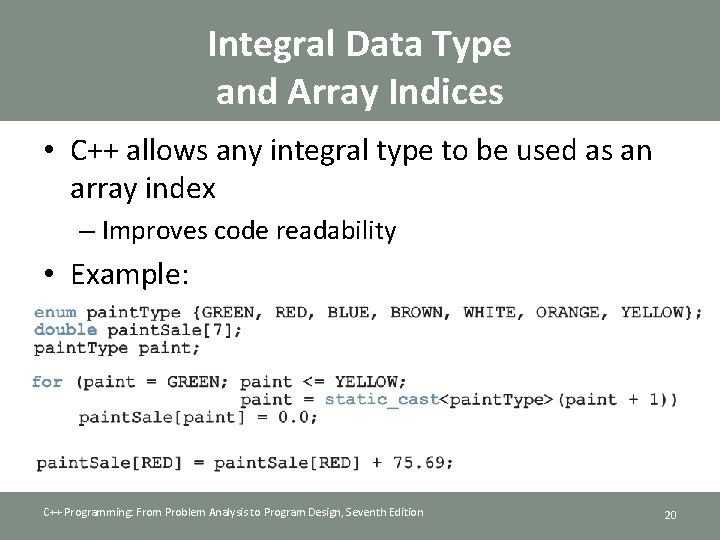Integral Data Type and Array Indices • C++ allows any integral type to be used as an array index – Improves code readability • Example: C++ Programming: From Problem Analysis to Program Design, Seventh Edition 20Other Ways to Declare Arrays • Examples: C++ Programming: From Problem Analysis to Program Design, Seventh Edition 21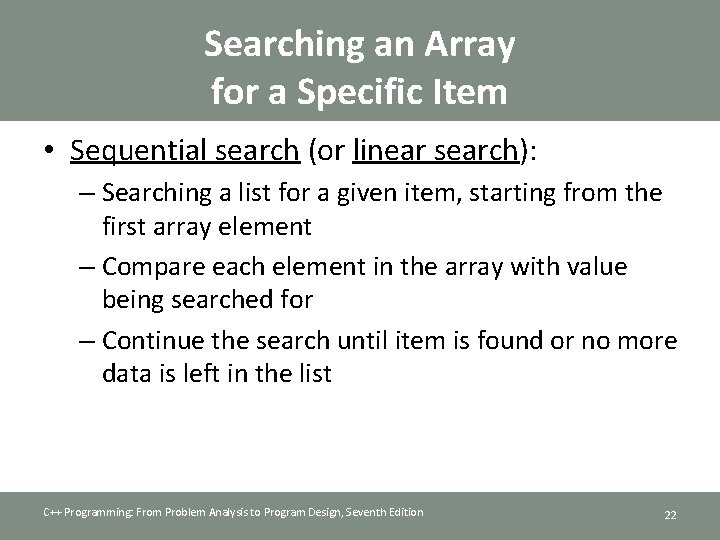Searching an Array for a Specific Item • Sequential search (or linear search): – Searching a list for a given item, starting from the first array element – Compare each element in the array with value being searched for – Continue the search until item is found or no more data is left in the list C++ Programming: From Problem Analysis to Program Design, Seventh Edition 22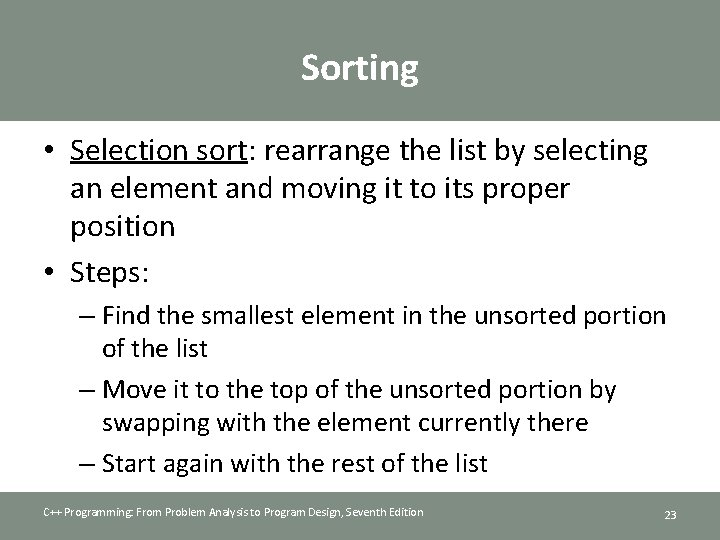Sorting • Selection sort: rearrange the list by selecting an element and moving it to its proper position • Steps: – Find the smallest element in the unsorted portion of the list – Move it to the top of the unsorted portion by swapping with the element currently there – Start again with the rest of the list C++ Programming: From Problem Analysis to Program Design, Seventh Edition 23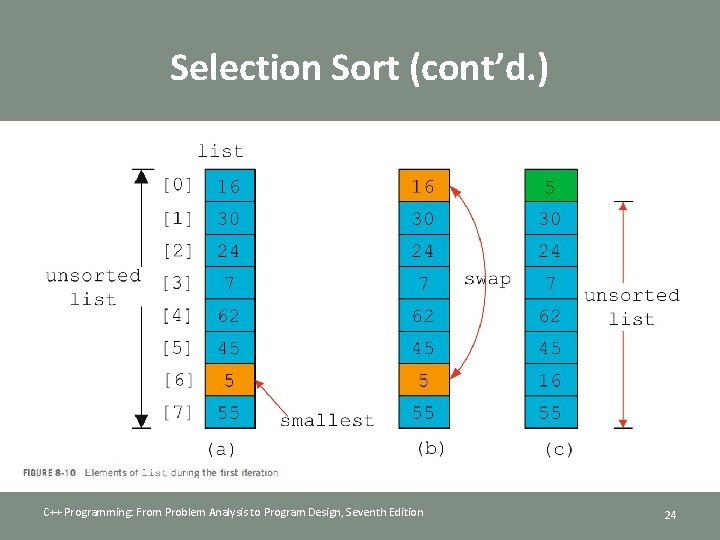Selection Sort (cont’d. ) C++ Programming: From Problem Analysis to Program Design, Seventh Edition 24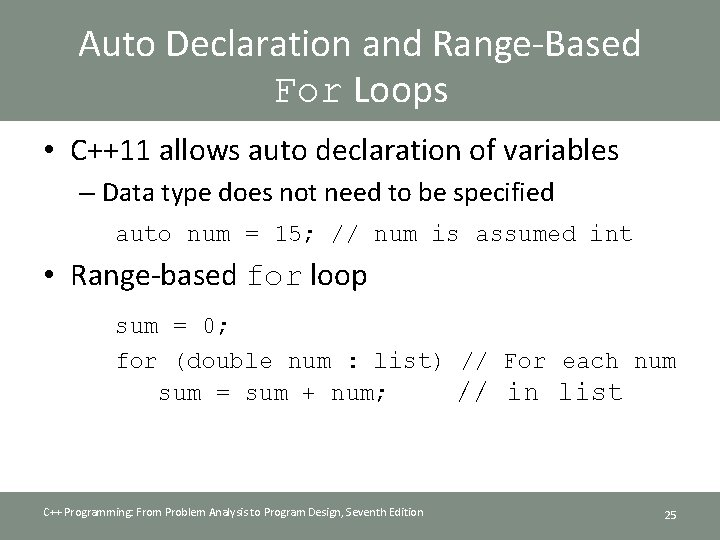Auto Declaration and Range-Based For Loops • C++11 allows auto declaration of variables – Data type does not need to be specified auto num = 15; // num is assumed int • Range-based for loop sum = 0; for (double num : list) // For each num sum = sum + num; // in list C++ Programming: From Problem Analysis to Program Design, Seventh Edition 25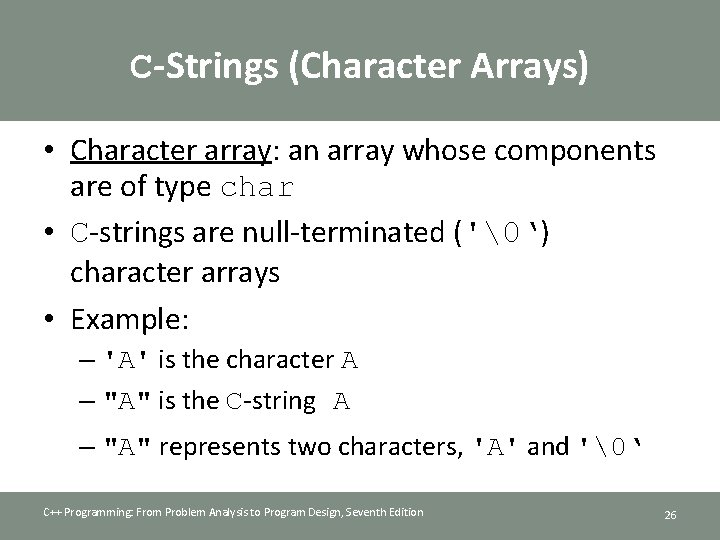C-Strings (Character Arrays) • Character array: an array whose components are of type char • C-strings are null-terminated ('‘) character arrays • Example: – 'A' is the character A – "A" is the C-string A – "A" represents two characters, 'A' and '‘ C++ Programming: From Problem Analysis to Program Design, Seventh Edition 26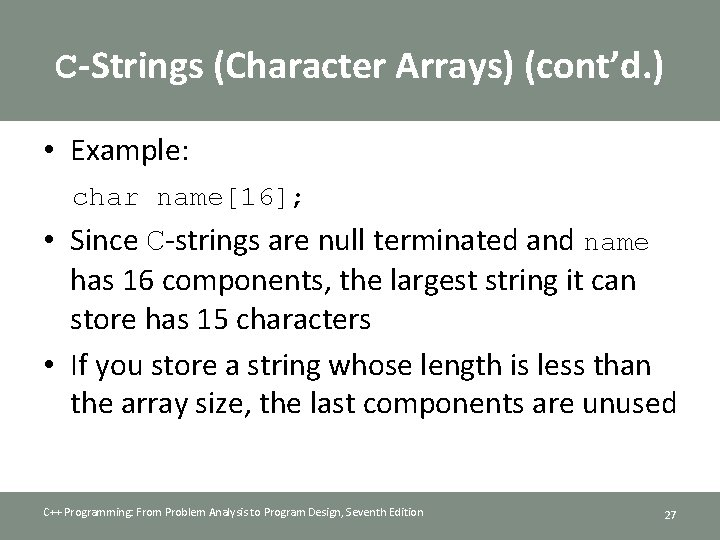C-Strings (Character Arrays) (cont’d. ) • Example: char name; • Since C-strings are null terminated and name has 16 components, the largest string it can store has 15 characters • If you store a string whose length is less than the array size, the last components are unused C++ Programming: From Problem Analysis to Program Design, Seventh Edition 27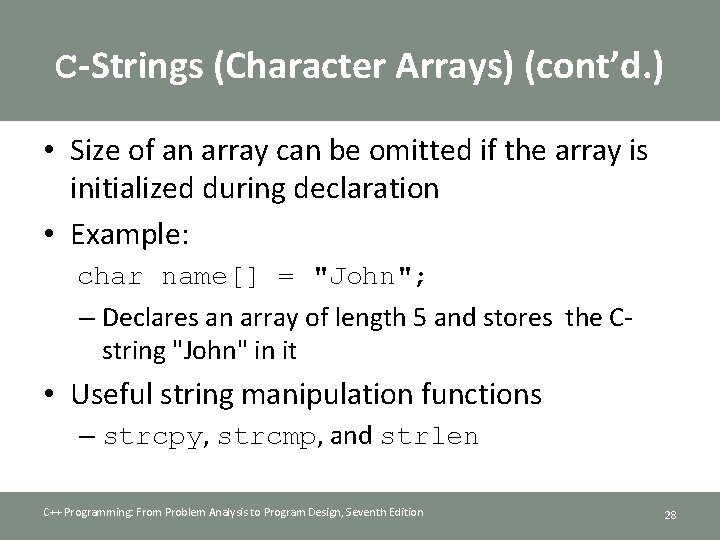C-Strings (Character Arrays) (cont’d. ) • Size of an array can be omitted if the array is initialized during declaration • Example: char name[] = "John"; – Declares an array of length 5 and stores the Cstring "John" in it • Useful string manipulation functions – strcpy, strcmp, and strlen C++ Programming: From Problem Analysis to Program Design, Seventh Edition 28String Comparison • C-strings are compared character by character using the collating sequence of the system – Use the function strcmp • If using the ASCII character set: – "Air" < "Boat" – "Air" < "An" – "Bill" < "Billy" – "Hello" < "hello" C++ Programming: From Problem Analysis to Program Design, Seventh Edition 29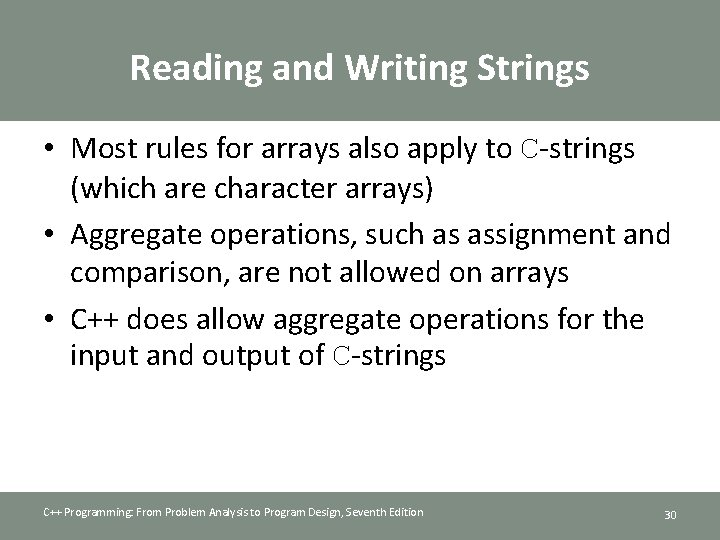Reading and Writing Strings • Most rules for arrays also apply to C-strings (which are character arrays) • Aggregate operations, such as assignment and comparison, are not allowed on arrays • C++ does allow aggregate operations for the input and output of C-strings C++ Programming: From Problem Analysis to Program Design, Seventh Edition 30String Input • Example: cin >> name; – Stores the next input C-string into name • To read strings with blanks, use get function: cin. get(str, m+1); – Stores the next m characters into str but the newline character is not stored in str – If input string has fewer than m characters, reading stops at the newline character C++ Programming: From Problem Analysis to Program Design, Seventh Edition 31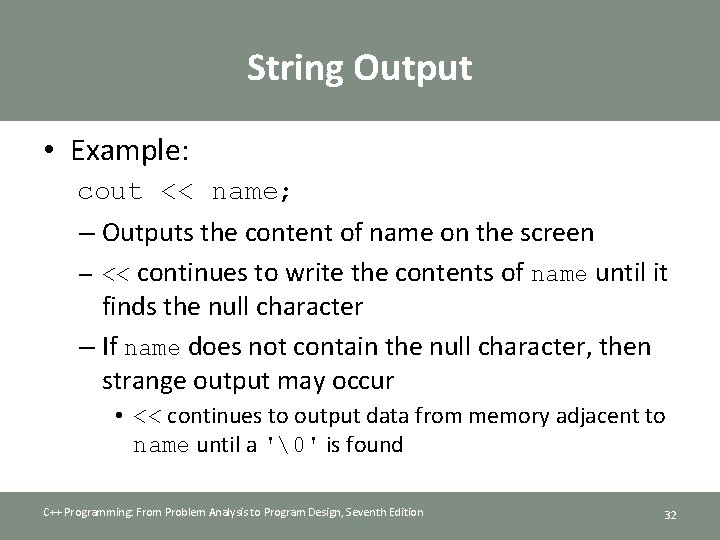String Output • Example: cout << name; – Outputs the content of name on the screen – << continues to write the contents of name until it finds the null character – If name does not contain the null character, then strange output may occur • << continues to output data from memory adjacent to name until a '' is found C++ Programming: From Problem Analysis to Program Design, Seventh Edition 32Specifying Input/Output Files at Execution Time • User can specify the name of an input and/or output file at execution time: C++ Programming: From Problem Analysis to Program Design, Seventh Edition 33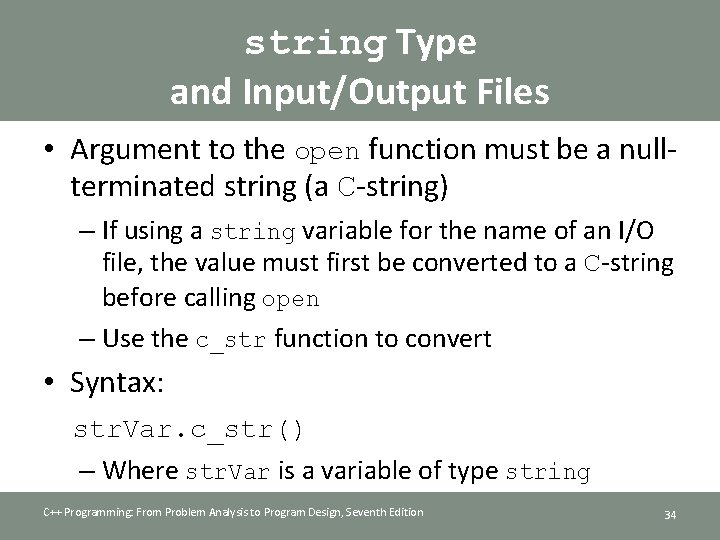string Type and Input/Output Files • Argument to the open function must be a nullterminated string (a C-string) – If using a string variable for the name of an I/O file, the value must first be converted to a C-string before calling open – Use the c_str function to convert • Syntax: str. Var. c_str() – Where str. Var is a variable of type string C++ Programming: From Problem Analysis to Program Design, Seventh Edition 34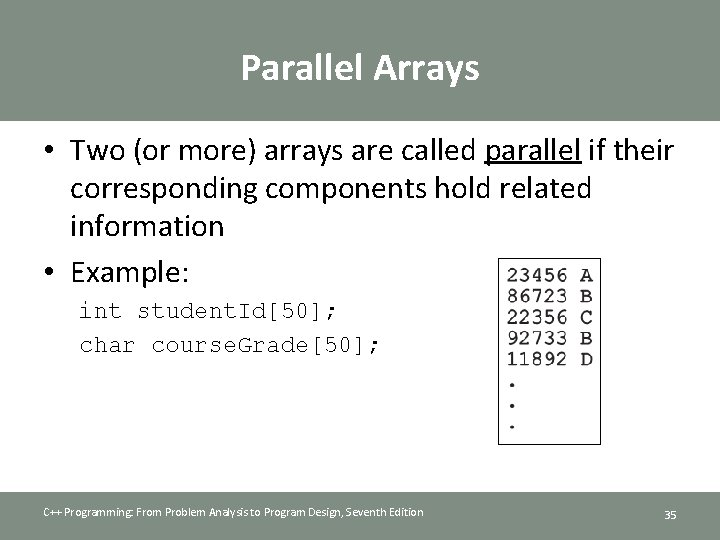Parallel Arrays • Two (or more) arrays are called parallel if their corresponding components hold related information • Example: int student. Id; char course. Grade; C++ Programming: From Problem Analysis to Program Design, Seventh Edition 35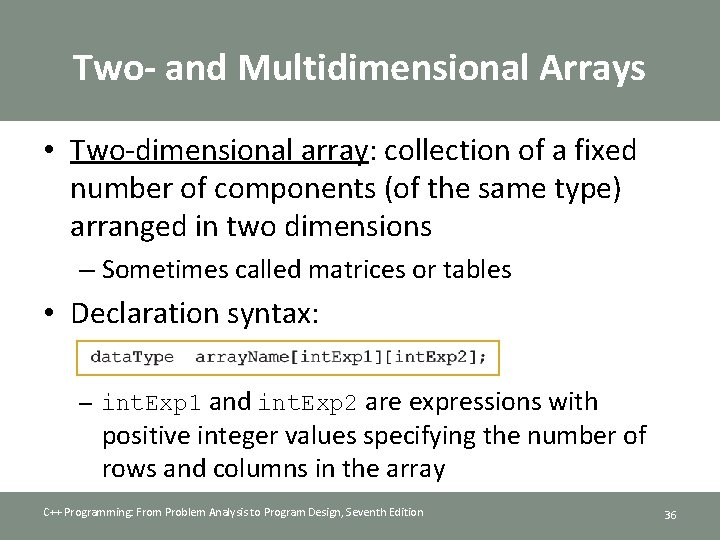Two- and Multidimensional Arrays • Two-dimensional array: collection of a fixed number of components (of the same type) arranged in two dimensions – Sometimes called matrices or tables • Declaration syntax: – int. Exp 1 and int. Exp 2 are expressions with positive integer values specifying the number of rows and columns in the array C++ Programming: From Problem Analysis to Program Design, Seventh Edition 36Accessing Array Components • Accessing components in a two-dimensional array: – Where index. Exp 1 and index. Exp 2 are expressions with positive integer values, and specify the row and column position • Example: sales = 25. 75; C++ Programming: From Problem Analysis to Program Design, Seventh Edition 37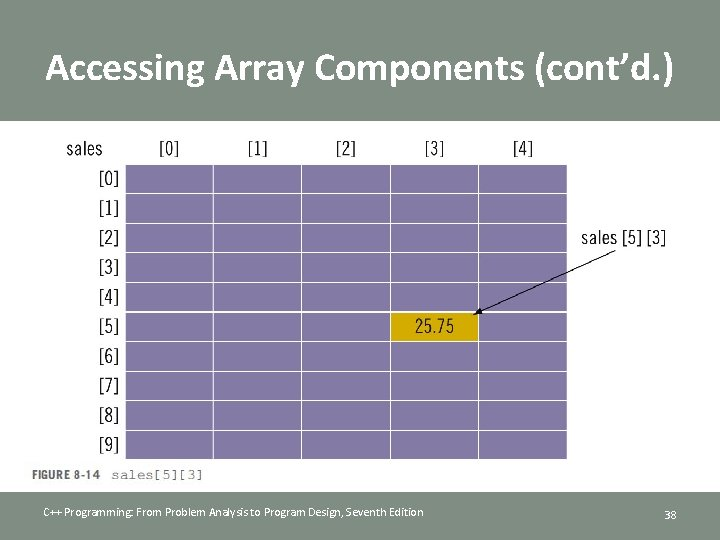Accessing Array Components (cont’d. ) C++ Programming: From Problem Analysis to Program Design, Seventh Edition 38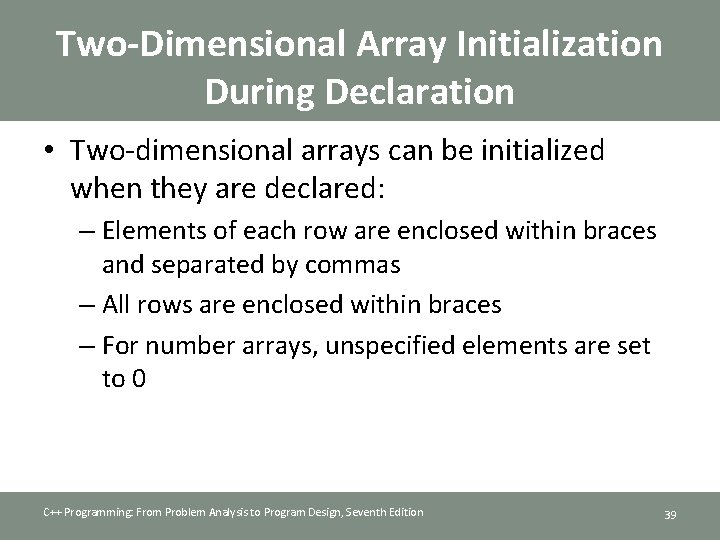Two-Dimensional Array Initialization During Declaration • Two-dimensional arrays can be initialized when they are declared: – Elements of each row are enclosed within braces and separated by commas – All rows are enclosed within braces – For number arrays, unspecified elements are set to 0 C++ Programming: From Problem Analysis to Program Design, Seventh Edition 39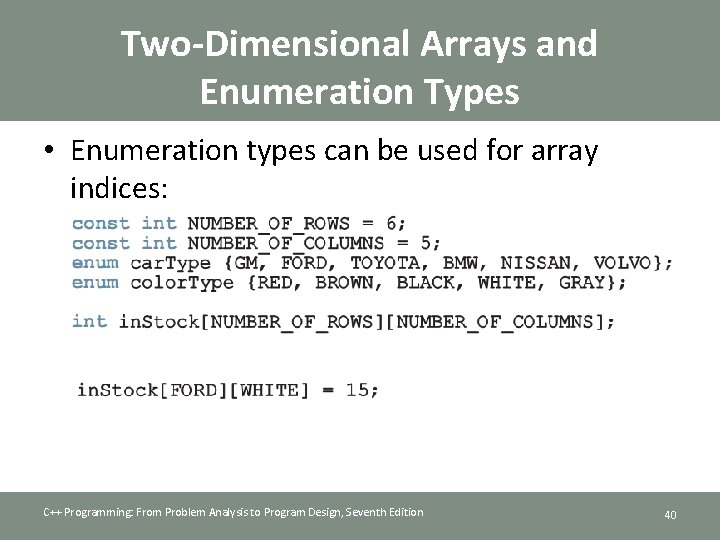Two-Dimensional Arrays and Enumeration Types • Enumeration types can be used for array indices: C++ Programming: From Problem Analysis to Program Design, Seventh Edition 40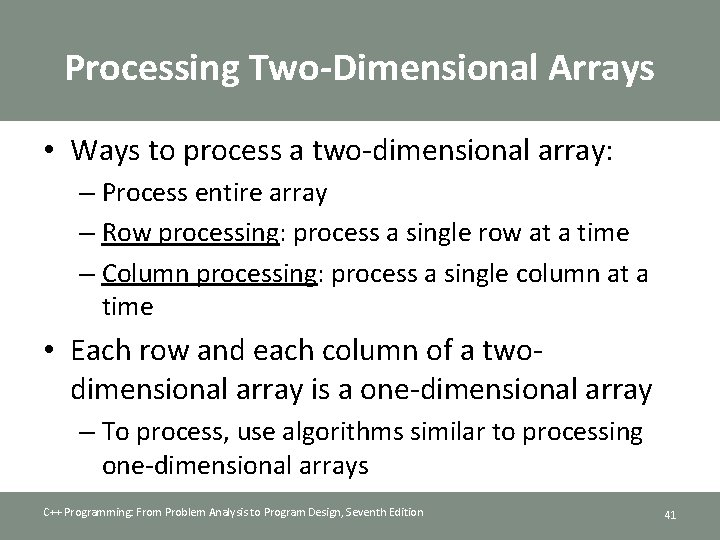Processing Two-Dimensional Arrays • Ways to process a two-dimensional array: – Process entire array – Row processing: process a single row at a time – Column processing: process a single column at a time • Each row and each column of a twodimensional array is a one-dimensional array – To process, use algorithms similar to processing one-dimensional arrays C++ Programming: From Problem Analysis to Program Design, Seventh Edition 41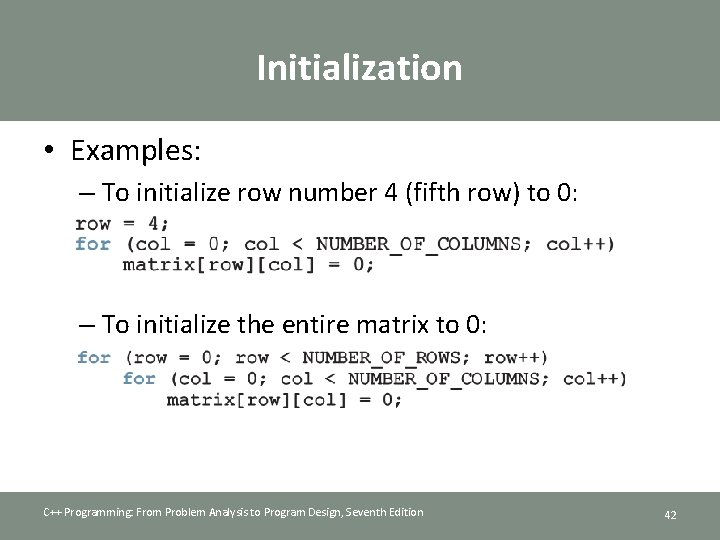Initialization • Examples: – To initialize row number 4 (fifth row) to 0: – To initialize the entire matrix to 0: C++ Programming: From Problem Analysis to Program Design, Seventh Edition 42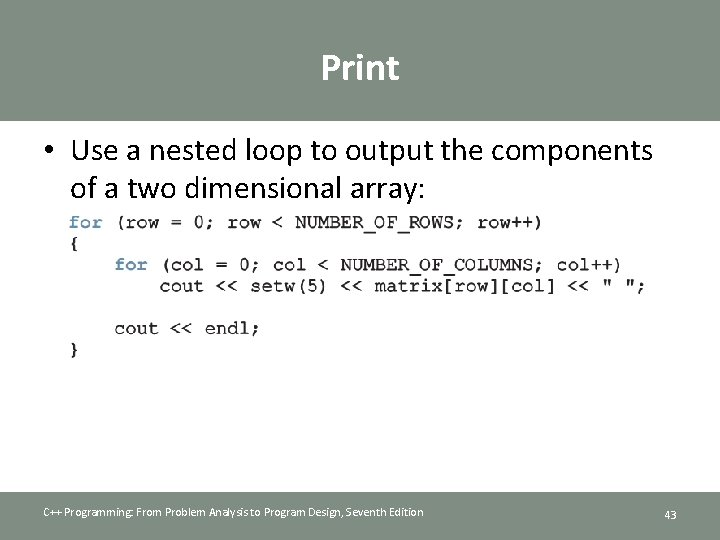Print • Use a nested loop to output the components of a two dimensional array: C++ Programming: From Problem Analysis to Program Design, Seventh Edition 43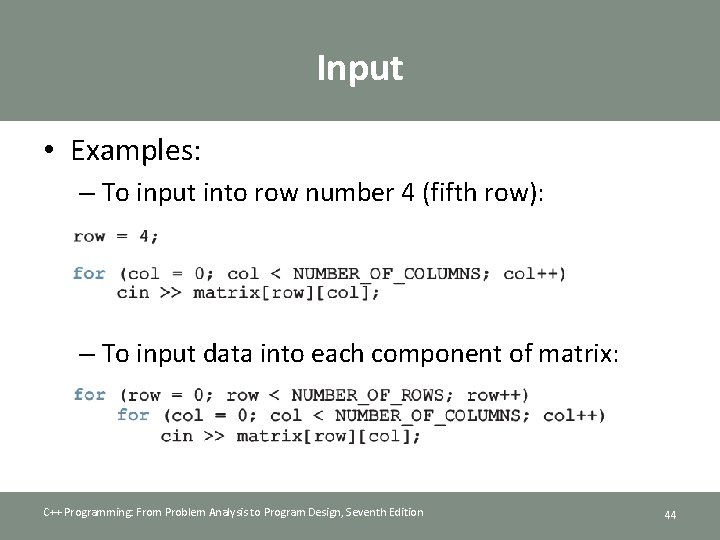Input • Examples: – To input into row number 4 (fifth row): – To input data into each component of matrix: C++ Programming: From Problem Analysis to Program Design, Seventh Edition 44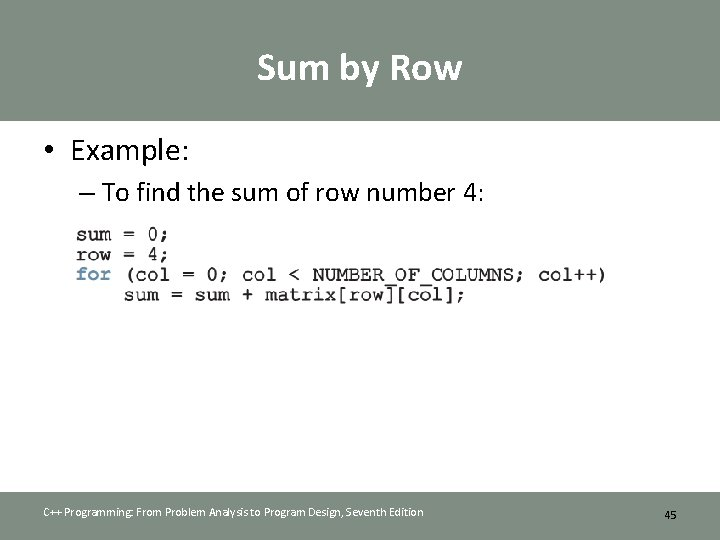Sum by Row • Example: – To find the sum of row number 4: C++ Programming: From Problem Analysis to Program Design, Seventh Edition 45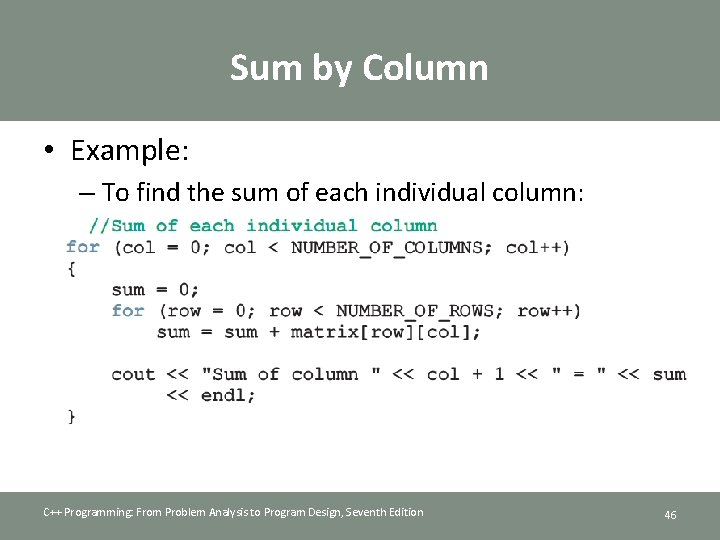Sum by Column • Example: – To find the sum of each individual column: C++ Programming: From Problem Analysis to Program Design, Seventh Edition 46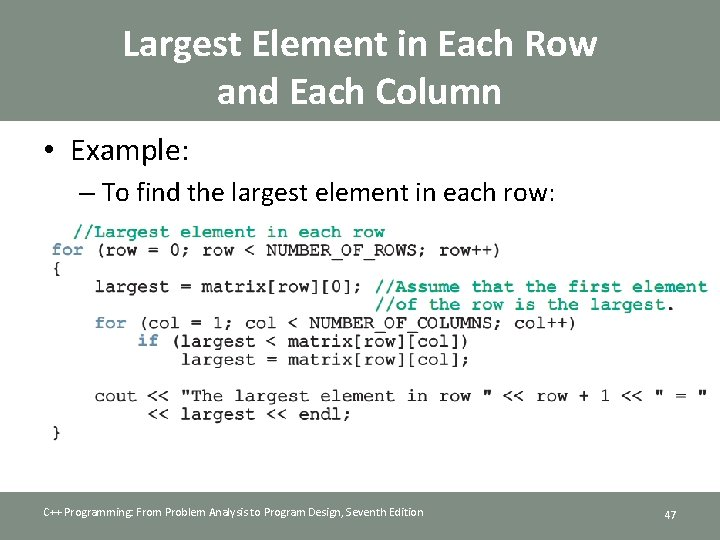Largest Element in Each Row and Each Column • Example: – To find the largest element in each row: C++ Programming: From Problem Analysis to Program Design, Seventh Edition 47Passing Two-Dimensional Arrays as Parameters to Functions • Two-dimensional arrays are passed by reference as parameters to a function – Base address is passed to formal parameter • Two-dimensional arrays are stored in row order • When declaring a two-dimensional array as a formal parameter, can omit size of first dimension, but not the second C++ Programming: From Problem Analysis to Program Design, Seventh Edition 48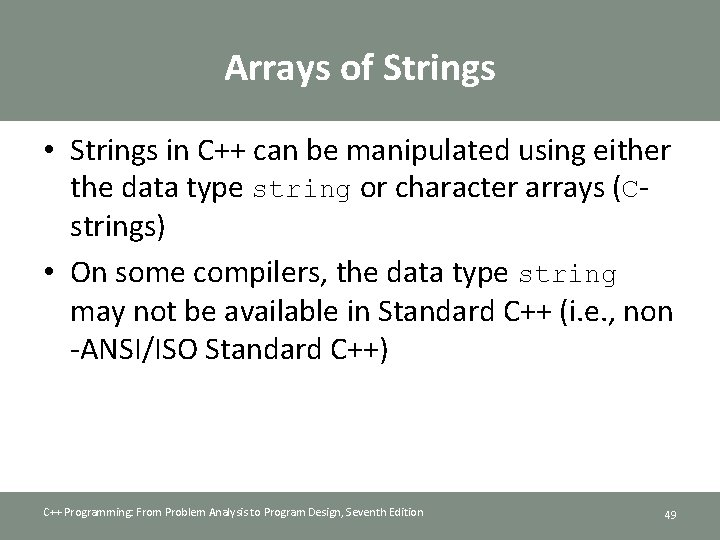Arrays of Strings • Strings in C++ can be manipulated using either the data type string or character arrays (Cstrings) • On some compilers, the data type string may not be available in Standard C++ (i. e. , non -ANSI/ISO Standard C++) C++ Programming: From Problem Analysis to Program Design, Seventh Edition 49Arrays of Strings and the string Type • To declare an array of 100 components of type string: string list; • Basic operations, such as assignment, comparison, and input/output, can be performed on values of the string type • The data in list can be processed just like any one-dimensional array C++ Programming: From Problem Analysis to Program Design, Seventh Edition 50Arrays of Strings and C-Strings (Character Arrays) C++ Programming: From Problem Analysis to Program Design, Seventh Edition 51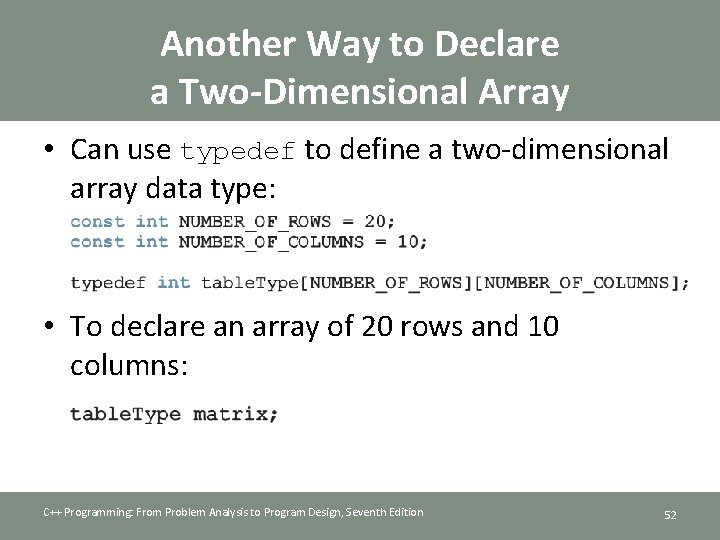Another Way to Declare a Two-Dimensional Array • Can use typedef to define a two-dimensional array data type: • To declare an array of 20 rows and 10 columns: C++ Programming: From Problem Analysis to Program Design, Seventh Edition 52Multidimensional Arrays • n-dimensional array: collection of a fixed number of elements arranged in n dimensions (n >= 1) • Declaration syntax: • To access a component: C++ Programming: From Problem Analysis to Program Design, Seventh Edition 53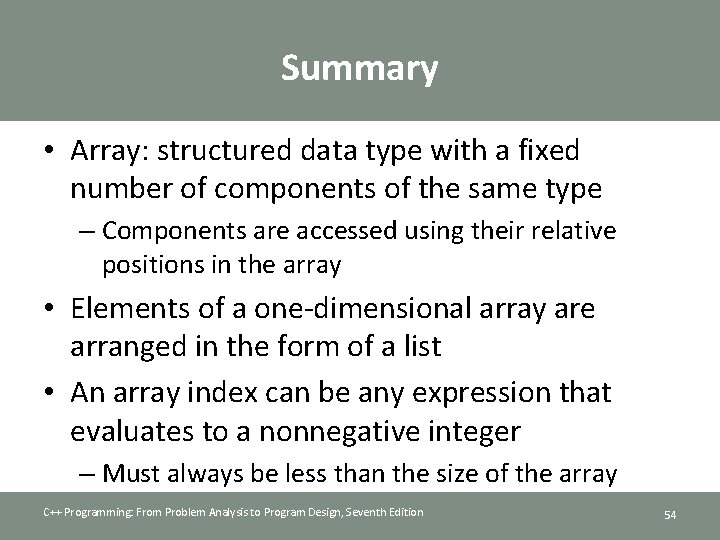Summary • Array: structured data type with a fixed number of components of the same type – Components are accessed using their relative positions in the array • Elements of a one-dimensional array are arranged in the form of a list • An array index can be any expression that evaluates to a nonnegative integer – Must always be less than the size of the array C++ Programming: From Problem Analysis to Program Design, Seventh Edition 54Summary (cont’d. ) • The base address of an array is the address of the first array component • When passing an array as an actual parameter, use only its name – Passed by reference only • A function cannot return an array type value • C++11 allows auto declaration of variables C++ Programming: From Problem Analysis to Program Design, Seventh Edition 55Summary (cont’d. ) • In C++, C-strings are null terminated and are stored in character arrays • Commonly used C-string manipulation functions include: – strcpy, strcmp, and strlen • Parallel arrays hold related information • In a two-dimensional array, the elements are arranged in a table form C++ Programming: From Problem Analysis to Program Design, Seventh Edition 56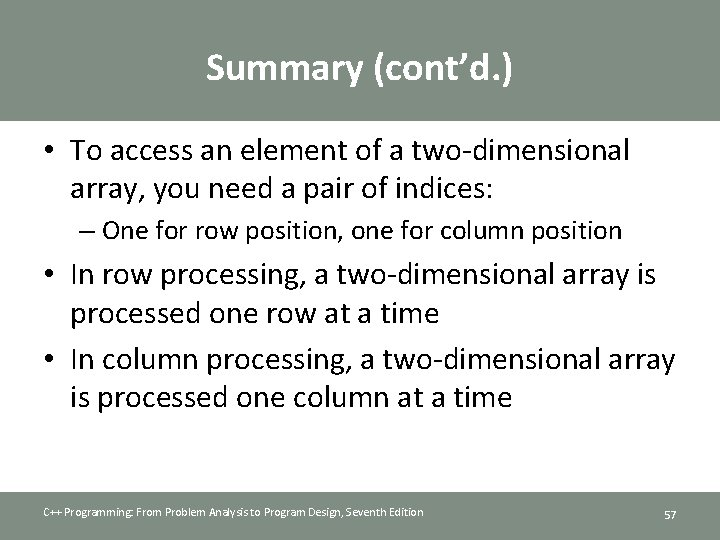Summary (cont’d. ) • To access an element of a two-dimensional array, you need a pair of indices: – One for row position, one for column position • In row processing, a two-dimensional array is processed one row at a time • In column processing, a two-dimensional array is processed one column at a time C++ Programming: From Problem Analysis to Program Design, Seventh Edition 57